161015 Epaper

 A Section B SectionC Section D Section161014 Epaper

 A Section B SectionC Section D Section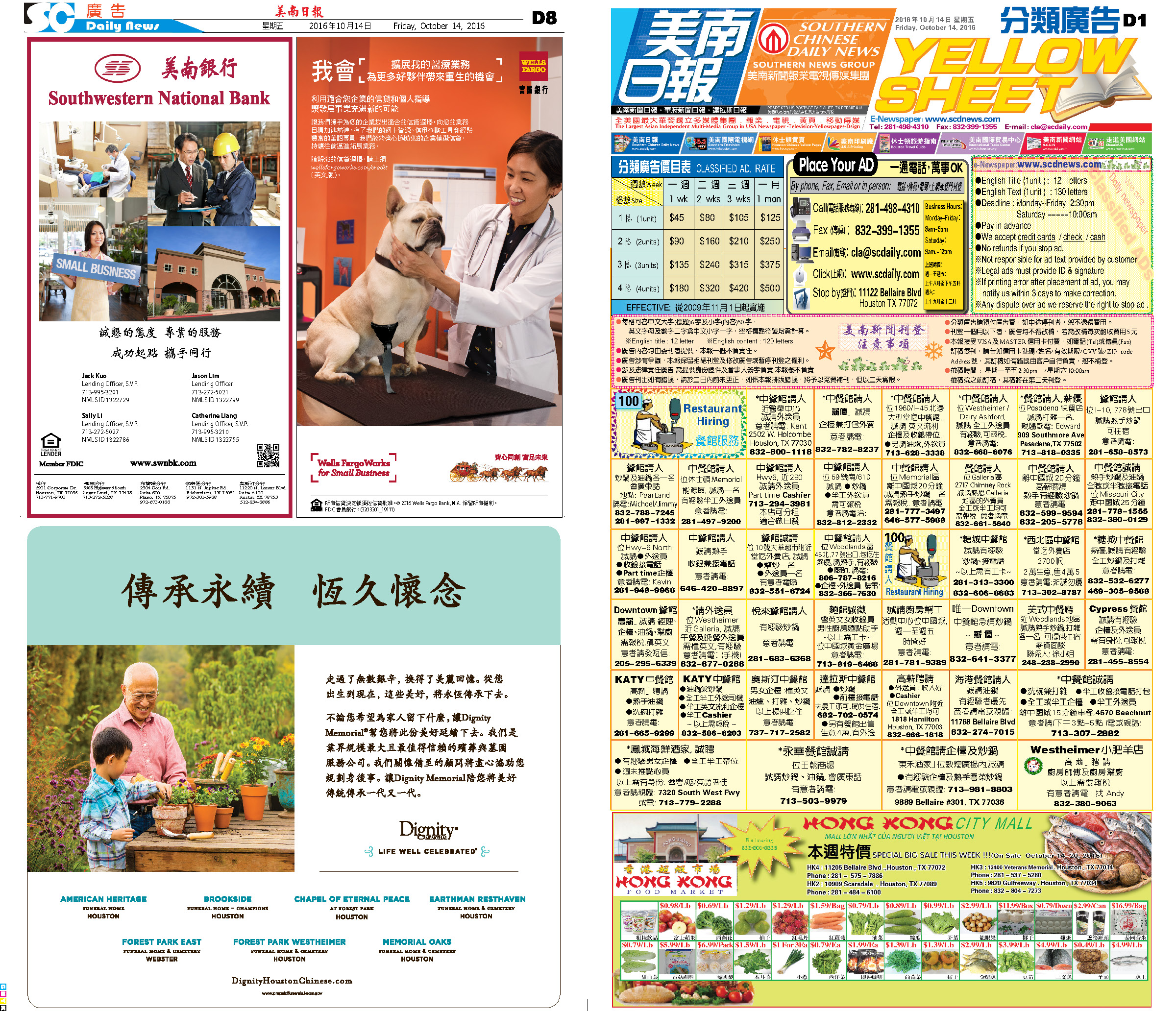161013 Epaper

 A Section B Section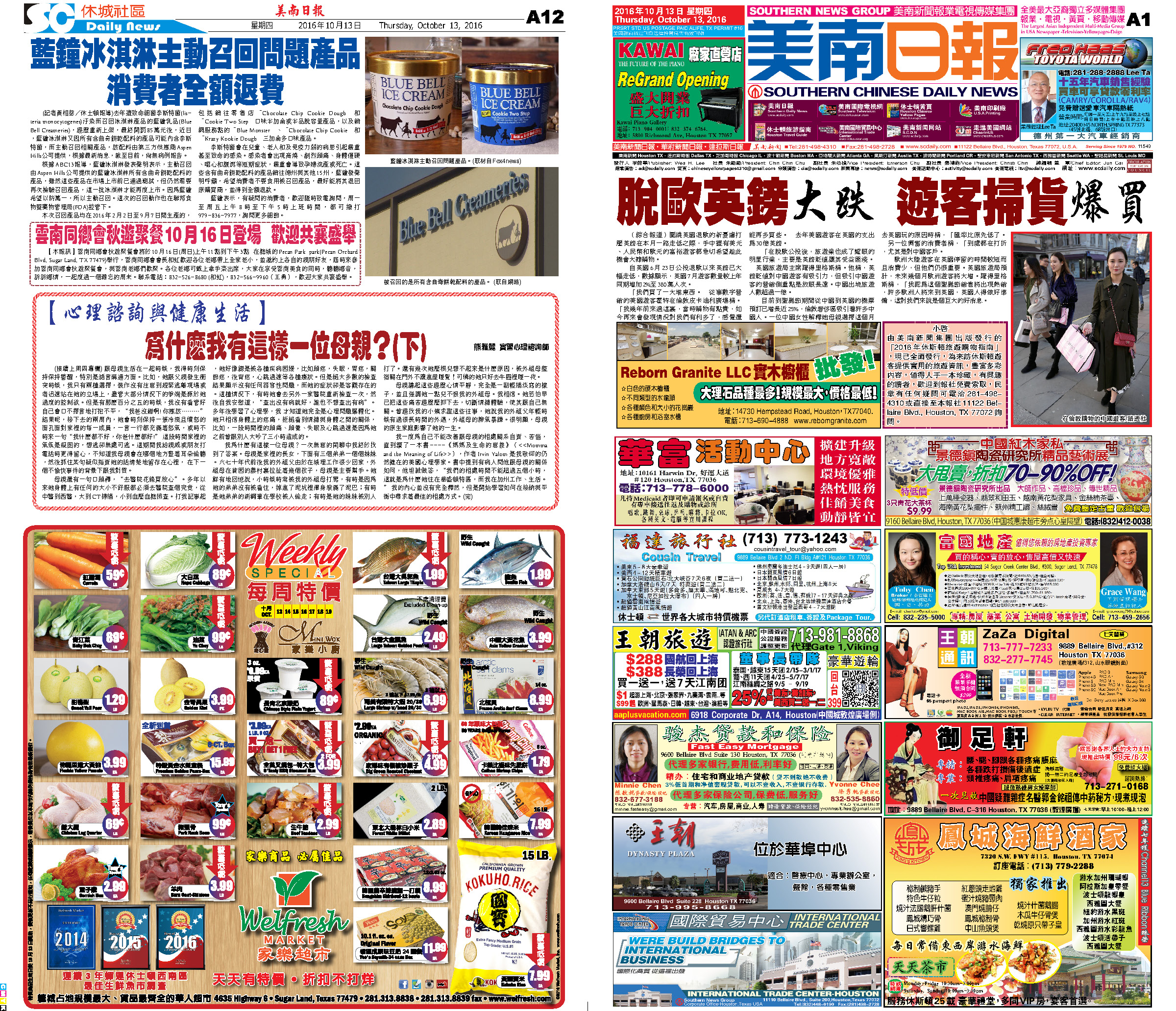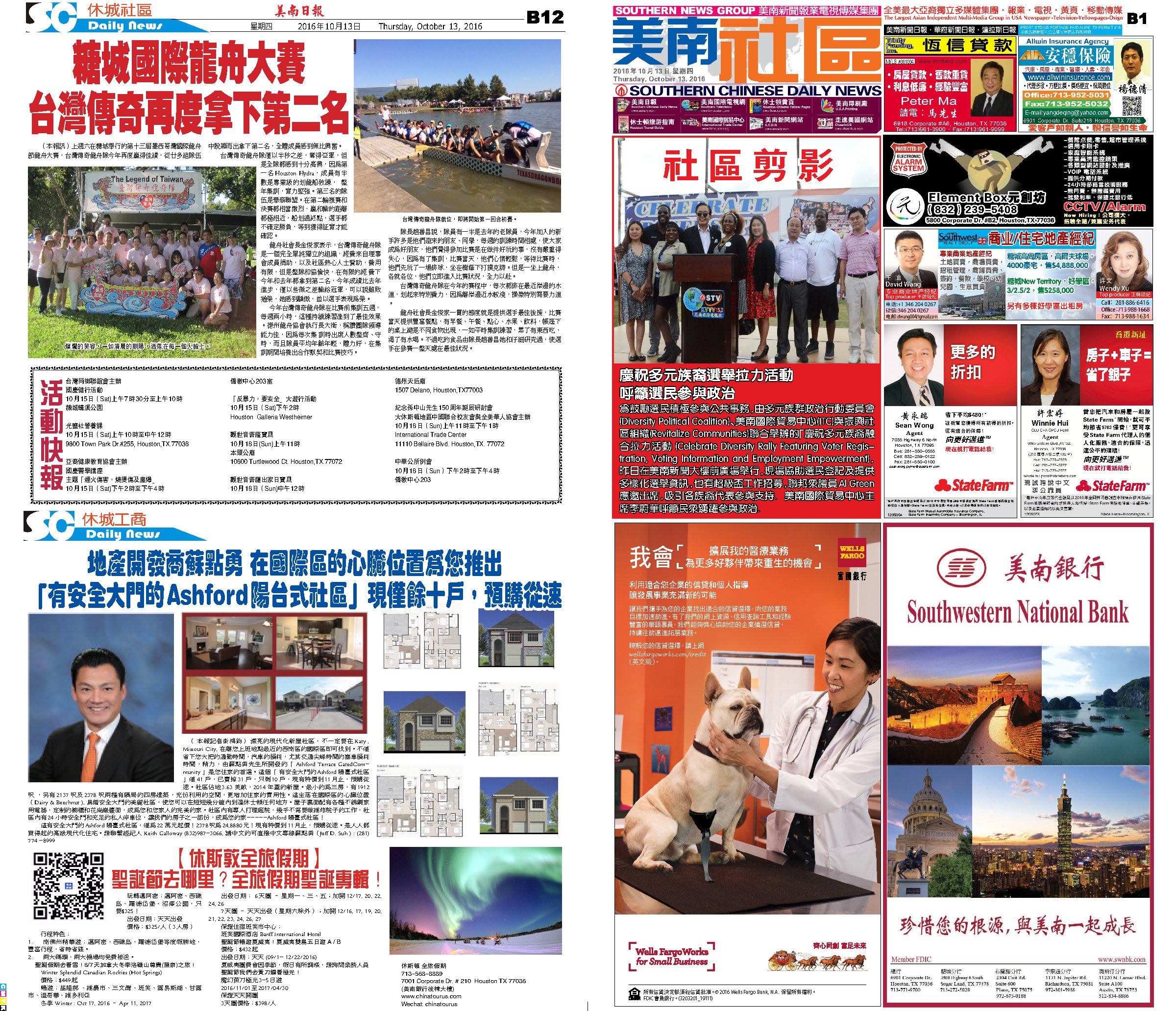C Section D Section161012 Epaper

 A Section B SectionC Section D Section161011 Epaper

 A Section B SectionC Section D Section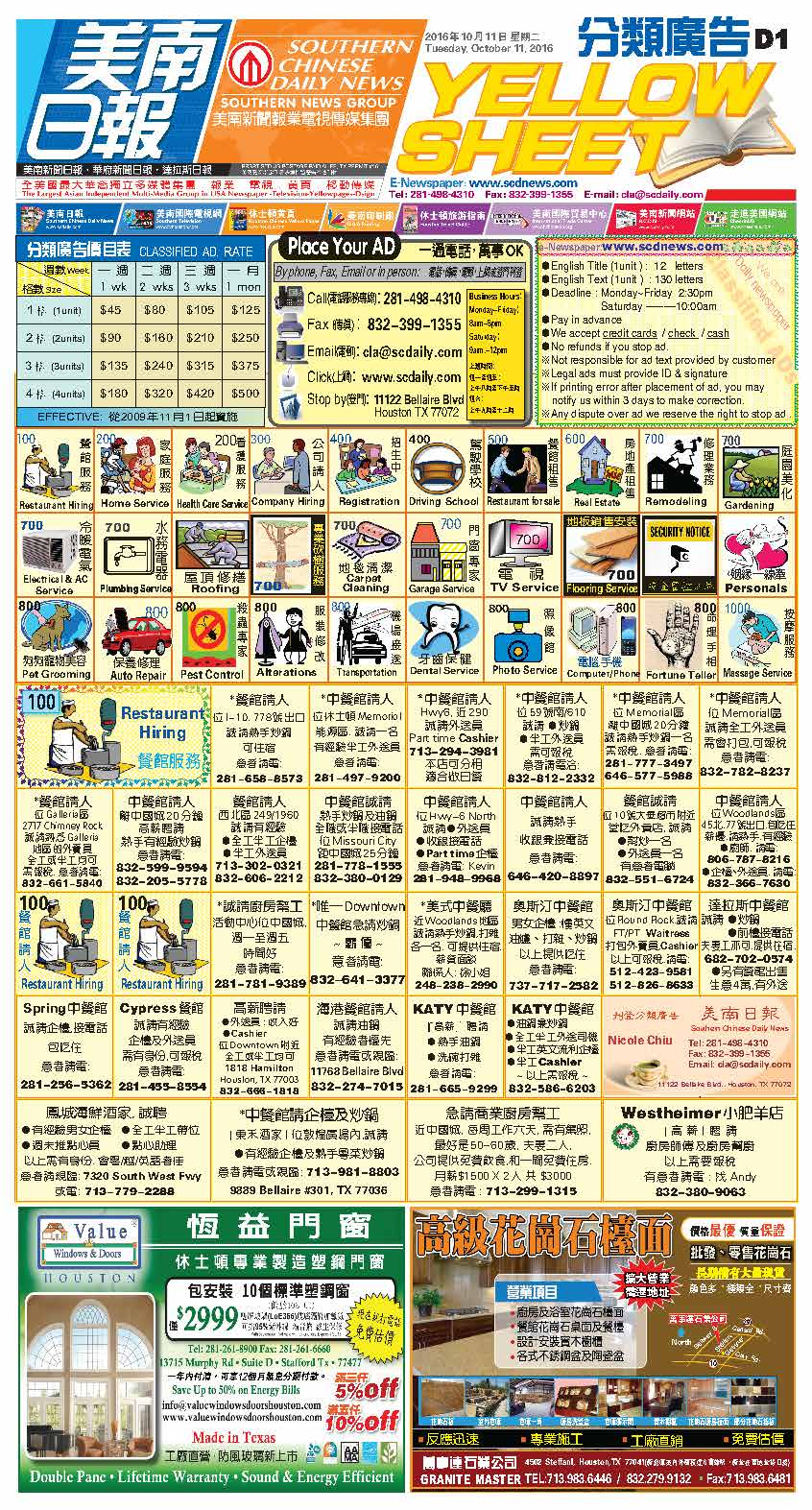161010 Epaper

 A Section B SectionC Section D Section161009 Epaper

 A Section B Section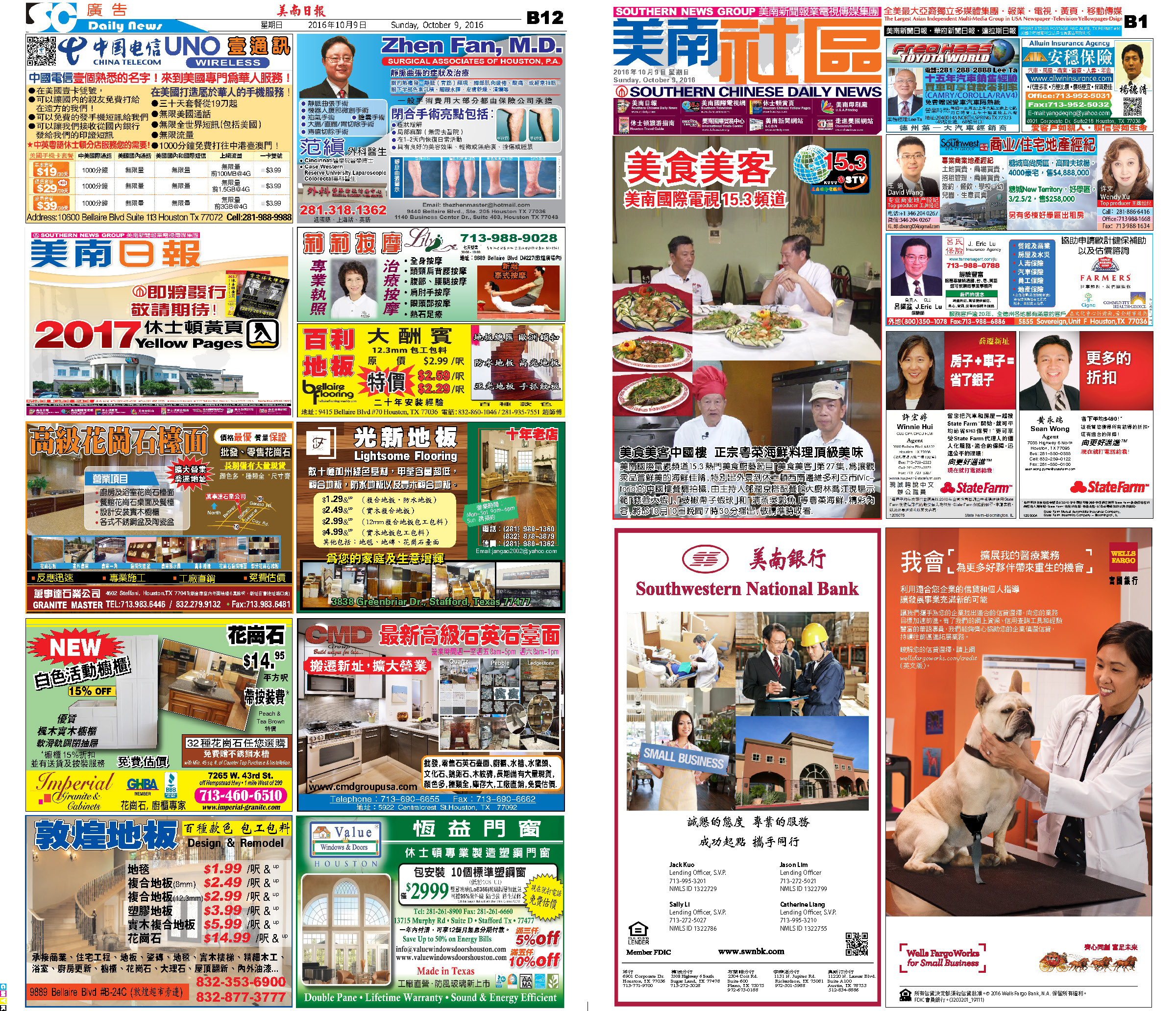C Section D Section161008 Epaper

 A Section B SectionC Section D Section161006 Epaper

 A Section B SectionC Section D Section161007 Epaper

 A Section B SectionC Section D Section161005 Epaper

 A Section B SectionC Section D Section